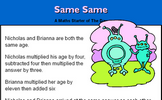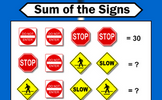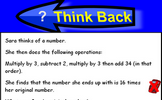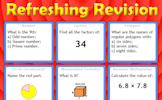# Representing solutions of equations and inequalities

### Term 1 starting in week 7 :: Estimated time: 3 weeks

• Understand the meaning of a solution
• Form and solve one-step and two-step equations (review)
• Form and solve one-step and two-step inequalities (review)
• Show solutions to inequalities on a number line
• Interpret representations on number lines as inequalities
• Draw straight line graphs (review)
• Find solutions to equations using straight line graphs
• Form and solve equations with unknowns on both sides
• Form and solve inequalities with unknowns on both sides
• Form and solve more complex equations and inequalities

For higher-attaining pupils:

• Represent solutions to inequalities using set notation
• Represent solutions to single inequalities on a graph
• Represent solutions to multiple inequalities on a graph
• Solve quadratic equations by factorisation
• Solve quadratic inequalities in one variable

This page should remember your ticks from one visit to the next for a period of time. It does this by using Local Storage so the information is saved only on the computer you are working on right now.

## Lesson Starters

Here are some suggestions for whole-class, projectable resources which can be used at the beginnings of each lesson in this block.

### 1st Lesson#### PYA

You have four minutes to write down as many equations as you can involving the given letters.

### 2nd Lesson#### Connecting Rules

Give 20 rules connecting x and y given their values.

### 3rd Lesson#### Lost Sheep

Which algebraic expression is the odd one out?

### 4th Lesson#### Less Than

This mathematics lesson starter invites pupils to interpret a three part algebraic inequality.

### 5th Lesson#### Mystic Maths

Work out why subtracting a two digit number from its reverse gives a multiple of nine.

### 6th Lesson#### Same Same

A problem involving two people's ages which can be solved using algebra.

### 7th Lesson#### Sum of the Signs

Each traffic sign stands for a number. Some of the sums of rows and columns are shown. What numbers might the signs stand for?

### 8th Lesson#### Think Back

A problem which can be answered by forming an algebraic equation then solving it.

### 9th Lesson#### Refreshing Revision

It is called Refreshing Revision because every time you refresh the page you get different revision questions.

Some of the Starters above are to reinforce concepts learnt, others are to introduce new ideas while others are on unrelated topics designed for retrieval practice or and opportunity to develop problem-solving skills.

White Rose ResourcesEnd of block assessments provide a quick progress check at the end of each block of learning to make sure students have understood the content covered. This Scheme of Learning was produced by White Rose Maths and is used here with permission granted on 30th June 2021.For All: